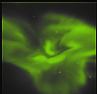Next: Resolution Up: Tomography Previous: Tomography   Contents

# Introduction

One of the main purposes of ALIS is to determine the full 3-D spatial structure of the aurora. This cannot be done with measurements from a single station and this is the main reason for developing ALIS as a multi-station system, with as many stations as possible to optimise the coverage of northern Scandinavia. This system gives simultaneous images of the aurora seen from different perspectives.

This chapter presents a short introduction to three-dimensional tomographic methods. Further it investigates the tomographic resolution of a ground-based system and the error sensitivity of such a system, and describes a general stopping criterion that gives a sufficient condition for when to interrupt iterative solutions for tomography.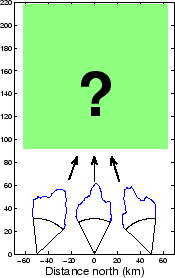Tomography is the method to determine the internal structure of an object starting from a set of images that in some way carry information of the integral intensity along the line-of-sight through the object. For x-ray tomography a known source radiates light from one side of the studied object and the image intensity acquired on the other side depends on the total absorption through the object along the ray path. For emission tomography, like auroral tomography, the images are made up of the total emission along the lines-of-sight. In Figure 3.1 a typical setup of a ground-based auroral tomographic problem is presented. Here there are three observation sites which record one-dimensional (1-D) images, and an unknown source to be determined. It is not evident that such inverse problems have solutions but for two-dimensional (2-D) tomography, Radon (1917) showed that it was possible to determine exactly the source function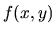. Hereis given in a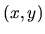-vertical plane, the measured intensities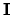, corresponding to our images, are given by line integrals(3.1)

where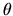and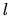determine the position of the detector and its line-of-sight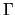, as defined in Figure 3.2 and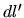is a length element along.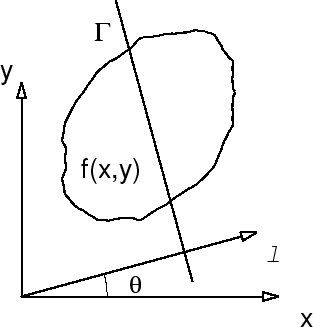It is possible to obtain an exact and unique solution of the source functionif and only if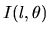is known for all raysthat cut the region where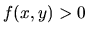. That is for an anglewe have to move the detector alongso that measurements are made for all rays that go through the object. The solution can be written as ( Gordon and Herman, 1974):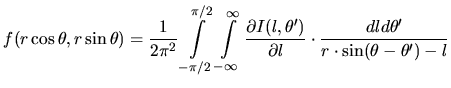(3.2)

For the three-dimensional tomographic problem exact solutions can be obtained if there is an observation point from which our image projections are made on every plane that cuts the region where the function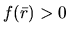( Kudo and Saito, 1990; Smith, 1990). There exist a number of analytical algorithms that determinefrom a set of image projections which closely satisfy the above condition ( Jacobsson, 1996; Grangeat, 1990).

However, in most cases these tomographic problems are ill-posed, meaning that they are sensitive to noise in the measurements or have either an infinite number of exact solutions or no exact solutions. With some regularization schemes, i.e. physical or mathematical constraints, the problem can, in a restricted sense, be transformed into a well-posed problem ( Ghosh Roy, 1991). Auroral and airglow tomography from ground-based multi-station measurements is ill-posed because only a few projections/images of the aurora are available and that the image data are all taken from below the object and thus are close to being linearly dependent.

The tomographic problem of ALIS is three-dimensional, and all performance, resolution and error analysis on the actual geometry suffers badly from the curse of dimensionality. That is, the total size of the tomographic problem is proportional to the total number of pixels in the images times the total number of volume elements (voxel = volume element, small cube, c.f. pixel = picture element) in the retrieved volume distribution. For a two-dimensional tomographic problem with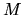voxels per side, the number of unknowns is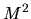projected onto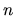images with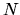pixels per one-dimensional image; the size of the problem is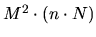. For a three-dimensional tomographic system the total number of pixels is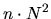and the number of voxels rises to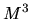, giving a total size of the problem of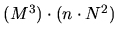. For systems studying the ionosphere the typical number of stations appears to be approximately 5 and typical number of pixels is 1000 per image dimension. For the retrieved volume distribution the number of voxels per side is of the order of 100. When we go from a two-dimensional to a three-dimensional tomographic problem the total size of the problem increases by a factor.

To simplify the analysis in this and the following two chapters, which describe the geometrical calibrations and the forward model, we will study a two-dimensional problem where emission distribution is to be determined in a vertical two-dimensional slice from one-dimensional images. This tomographic model system makes one-dimensional image measurements with the imaging characteristics of the ALIS cameras from four ground-based stations separated by 50 km from each other. The principles of tomographic inversion and all the characteristics of ground-based auroral tomography remain in this 2-D problem but the size is small enough to allow use of the most rigorous analysis of its resolution and retrieval characteristics.

Since ALIS currently only has six ground-based stations, and thus is very far from fulfilling the necessary requirements for obtaining exact reconstructions, we turn to approximate methods in order to obtain the best solution possible.

In order to be able to solve the tomographic problem it is necessary to have good knowledge about the forward problem or forward model. That is, we must know what fraction of the photons emitted in a volume element will be detected and where in the image the intensity will appear. In this model case we have to know how the auroral distribution in a slice is projected down onto the images, as depicted in Figure 3.3.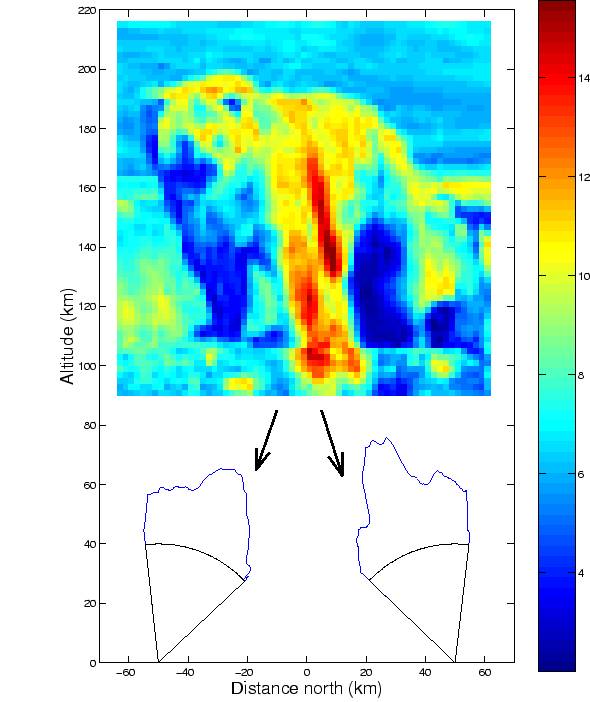The mathematical notation for this is: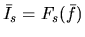(3.3)

where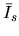is the image from stations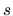,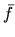is the two-dimensional source function and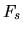is the forward model for station. Here we will not go into the details of the forward model; it is sufficient to note that it is important to know the pixel lines-of-sight and the sensitivities of the cameras. Further it is necessary to note that the cameras used by ALIS have a linear response to intensity and that the emissions currently measured by ALIS are all optically thin which implies that the forward model in equation (3.3) can be simplified to a matrix multiplication: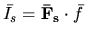(3.4)

where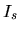is the image from stationandis the transfer matrix from the voxel spaceto the image.

If we solve the tomographic problem analytically, we will encouner the problem that the forward model is not a deterministic process, but rather a random process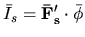(3.5)

where a component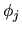of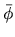is a sample from one random variable with a probability distribution function that depends on several processes from the emission of photons in voxel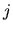to the detection in the image. Neglecting the randomness in all processes from the camera to the voxel, the random direction of photon emission remains. Since the distribution of emission can safely be assumed to be isotropic and the photon emissions are independent from each other, the probability distribution for the number of photons emitted towards the camera is a binomially distributed random variable: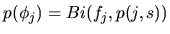(3.6)

where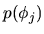is the probability of gettingphotons,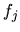is the total number of photons emitted in voxeland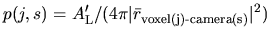(3.7)

is the probability that a photon is emitted towards the front lens of camera,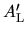is the area of the front lens as seen from voxel. With respect to work on aurora for which the number of photons emitted is large and the probability is small, the binomial distribution in equation (3.6) is well approximated by a normal distribution: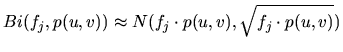(3.8)

Thus we see that the imaging process is a stochastic one for which the probability of getting a set of measured imagesfrom a source distributionis:(3.9)

where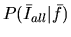is the conditional probability of getting the measured images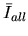given the source distribution. For auroral imaging with ALIS the image intensity has a probability distribution that is approximately: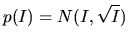(3.10)

The solution to the inverse problem is then to find the source distribution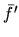that is most probable given the measurementsand considering equation (3.9) and Bayes' rule the resulting problem is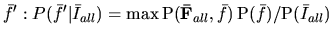(3.11)

This is the maximum likelihood solution of the inverse problem. For this case in which the random processes have normal distributions the maximum likelihood solution is the well-known least square solution. For the three-dimensional inverse problem the system transfer matrix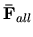is too large to invert directly, so we use iterative methods to solve the set of linear equations.

The simple and robust iterative methods we have chosen are the algebraic reconstruction technique (ART) and the simultaneous iterative reconstruction technique (SIRT) ( Gordon and Herman, 1974). The working principle of ART is then to take an initial guess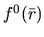and project that guess down to an image by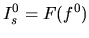(3.12)

Then the current solutionis updated voxel by voxel according to: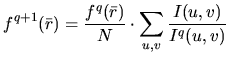(3.13)

This is a modified multiplicative ART update where the update is a weighted average of the ratios of the measured image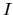andfor the pixelswhose lines-of-sight intersect the voxel at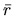.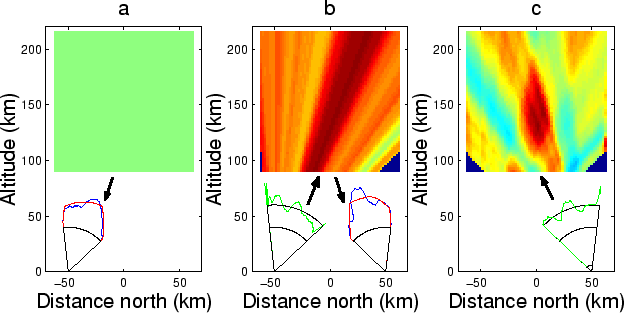The initial progress of this iterative reconstruction scheme is shown in Figure 3.4. First a constant initial guess is projected down onto one image. Then the ratio between the measured image and the projection of the start guess is calculated. This ratio is then projected out into the solution. In this way the voxel values along a pixel line-of-sight is increased if the measure image is larger than the projection from the start guess and vice-verse. This iteration is then proceeded over all stations in random order until a stopping criteria is encountered.

ART is a reconstruction scheme that converges fast but is sensitive to noise in the images ( Aso et al., 1993). A modified version is SIRT for which the current guess/solutionis projected down to all stations at once and the voxel intensity in the reconstruction is updated with the average ratio of pixels from all stations.

It is possible to use a priori knowledge about the aurora, such as field-alignedness of the auroral structures ( Semeter, 1997; Aso et al., 1990), in the reconstruction process in order to bias the reconstruction and to avoid noise amplification thereby stabilising the solution. Several more general constraints can also be used to reduce the effect of noise in the images, e.g. spatial low pass filtering in 3-D as outlined in paper I, horizontal median filtering, horizontal smoothing of field aligned intensity variation Aso et al. (1993).

Several more advanced iterative schemes exist and the technically interested reader is directed to Veklerov and Llacer (1989b).Next: Resolution Up: Tomography Previous: Tomography   Contents

copyright Björn Gustavsson 2000-10-24